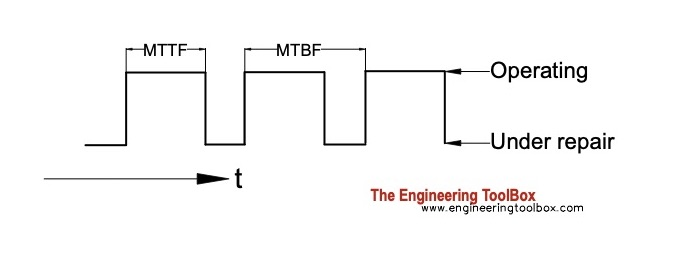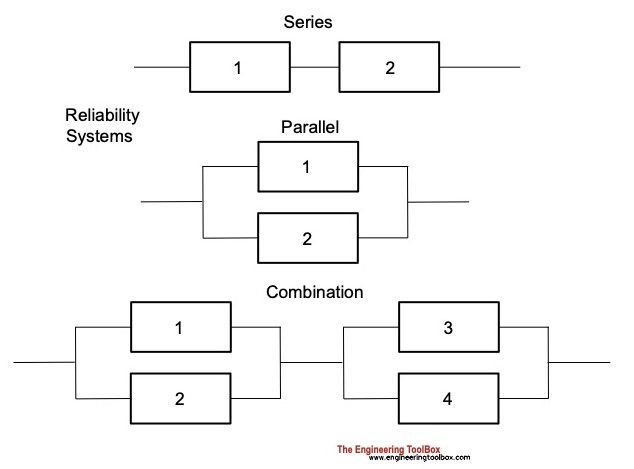Engineering ToolBox - Resources, Tools and Basic Information for Engineering and Design of Technical Applications!

# Reliability of Machine Components

## Mean Time Between Failure - MTB and reliability of machine components and systems.

Reliability characterizes components or system of components by the probability they will perform the desired functions for a given time.

In general -

• more components and/or more complicated systems reduces reliability
• simpler systems with few components increases reliability

Reliability equations:

### Reliability

Reliability at a given time:

R = e-λt                                (1)

where

R = reliability. Values between 0 - 1 where value 1 indicates 100% live components and value 0 indicates 0% live components.

λ = proportional failure rate - a failure rate expressed as a proportion of initial number of live components - No - at time t

t = time (hours) - Note! other units can be used as long as the use is consistent through the calculations.

The failure rate at time t can be expressed as

λ = NF / (No t)

= (No - Ns) / (No t)                 (2)

where

NF = No - Ns = number of failing components at time t

Ns = number of live surviving components at time t

No = initial number of live components at time zero

#### Example - Failure Rate and Reliability

A car manufacturer sells 400000 cars of a certain model in one year. During the the first tree years the owners of 50000 of these cars experience major failures. The failure rate can be calculated as

λ = (50000 cars)/ ((400000 cars) (3 years))

= 0.042 (per year)

The reliability of a model of this car within three years can be calculated as

R = e-(0.042 1/year) (3 year)

= 0.88

= 88 %

### Unreliability - the Probability for a Device to Fail

The connection between reliability and unreliability:

R + Q = 1                              (3)

where

Q = unreliability. Values between 0 - 1 where value 1 indicates 0% live components and value 0 indicates 100% live components.

(1) and (2) can be used to express unreliability

Q = 1 -e-λt                               (4)

#### Example - Unreliability

The unreliability of the car model in the example above within three years can be calculated as

Q = 1 - e-(0.042 1/year) (3 year)

= 0.12

= 12 %

### Number of Live Components

The number of live surviving components in a system at a given time:

Ns = No e-λt                            (5)

### Number of Failure Components

The number of failure dead components in a system at a given time:

Ns = No (1 - e-λt)                        (6)

### Mean Time Between Failures - MTBF

Mean time between failures - MTBF:

MTBF = 1 / λ                         (7)

where

MTBF = Mean Time Between Failure (hours)

• MTTF - Mean Time To Failure is an alternative to MTBF#### Example - Mean Time Between Failure

From the example above the failure rate is 0.42 per year. MTBF can be calculated as

MTBF = 1 / (0.042 1/year)

= 23.8 years

Mean Time Between Failure (MTBF) can be determined by rating Total Surviving Hours against Number of Failures as

MTBF = ts / NF                      (8)

where

ts = total surviving hours (hours)

Combining (5) with the formulas for reliability and more

R = e-t/m                        (1b)

Q = 1 -e-t/m                       (4b)

Ns = No e-t/m                      (5b)

Nf = No (1 - e-t/m)                     (6b)

### MTBF vs. MTTF vs. MTTR

• MTBF - Mean Time Between Failures > commonly used to determine average time between failures
• MTTF - Mean Time To Failure > commonly used for replaceable products that cannot be repaired
• MTTR - Mean Time To Repair > commonly used to determine how long it will take to get a failed product running again

MTBF and MTTF are often used interchangeably.

### Systems Reliability

There are two basic types of reliability systems - series and parallel - and combinations of them.

• in a series system - all devices in the system must work for the system to work
• in a parallel system - the system works if at least one device in the system works#### Reliability of Systems in Series

Reliability of systems in series can be expressed as

Rs = R1 R2                        (9)

where

Rs = system reliability

R1,2 = subsystem reliability

##### Example - Reliability of Systems in Series

From the example above the reliability of a car over three year is 0.88. If we depends on two cars for a mission - whatever it is - the reliability for our mission will be

Rs = 0.88 0.88

= 0.77

= 77 %

#### Reliability of Systems in Parallel

Reliability of systems in parallel can be expressed as

Rs = R1 Q2 + R2 Q1 + R1 R2                       (10)

where

Q1,2 = (1 - R1,2) subsystem unreliability

##### Example - Reliability of Systems in Parallel

From the example above the reliability of a car over three year is 0.88. If it is enough with one of our two cars for our mission - the reliability for our mission can be calculated as

Rs = 0.88 (1- 0.88) + 0.88 (1- 0.88) + 0.88 0.88

= 0.99

= 99 %

Note! - two systems in parallel with 99 % reliability vs. one system alone with reliability 88 % shows the power of redundancy.

## Related Topics

• ### Risk, Reliability and Safety

Risk, reliability and safety in process control systems.

## Related Documents

• ### Alternating Pumps

Securing a system and achieve equal wear by alternating pumps in parallel.
• ### DFA - Design for Assembly index

Design for Assembly Index indicates how easy it is to assembly a component.
• ### High Pressure Steam Systems - Safety Valve Capacities

Sizing safety valves according boiler output power in high pressure systems (kW and Btu/hr)
• ### NDT - Non Destructive Testing

Non-destructive construction testing.
• ### Piston Engines - Compression Ratios

Cylinder volume and compression ratios in piston engines.
• ### Steels - Endurance Limits and Fatigue Stress

Endurance limits and fatigue stress for steels.

## Search

Search is the most efficient way to navigate the Engineering ToolBox.

## Engineering ToolBox - SketchUp Extension - Online 3D modeling!

Add standard and customized parametric components - like flange beams, lumbers, piping, stairs and more - to your Sketchup model with the Engineering ToolBox - SketchUp Extension - enabled for use with older versions of the amazing SketchUp Make and the newer "up to date" SketchUp Pro . Add the Engineering ToolBox extension to your SketchUp Make/Pro from the Extension Warehouse !

We don't collect information from our users. More about

## Citation

• The Engineering ToolBox (2005). Reliability of Machine Components. [online] Available at: https://www.engineeringtoolbox.com/reliability-d_953.html [Accessed Day Month Year].

Modify the access date according your visit.

12.8.9